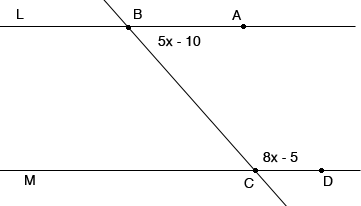On my math homework I have a problem where it says find the value of x so that L is parallel to M on one of the there are three lines. the top one is horizontal which is L. the bottom one is also horizontal directly under L which is M. there is another line that is diagonal going through the middle sections of L and M. To the right of the diagonal line and right under line L is an equation 5x-10 to the right of the diagonal line and right above line M is an equation 8X-5 I tried to find what X is but I can't because it never matches both equations no matter what way I try to do it. Hi Barbara, Here is what I think you are describing. I put some points on the lines and labeled them.I think that 5x - 10 is the measure of the angle ABC and 8x - 5 is the measure of the angle BCD. What do you know about these angles if L and M are parallel? Penny